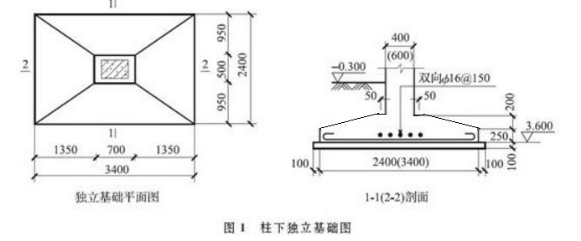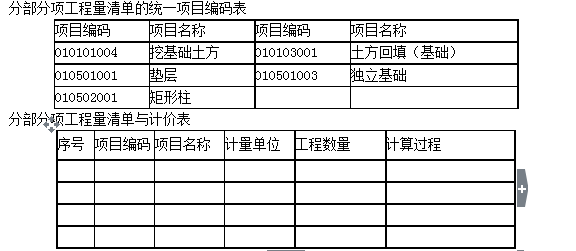# 造价工程师《案例分析》土建题

01)根据图示内容和《建设工程工程量清单计价规范》的规定，根据分部分项工程量清单的统一项目编码所列清单项目编制±0.00以下的分部分项工程量清单，并将计算过程及结果填入“分部分项工程量清单与计价表”。2)某承包商拟投标该工程，根据地质资料，确定柱基础为人工放坡开挖，工作面每边增加0.3m;自垫层上表面开始放坡，放坡系数为0.33;基坑边可堆土490m3;余土用翻斗车外运200m。该承包商使用的消耗量定额如下：挖1m3土方，用工0.48工日(已包括基底钎探用工);装运(外运200m)1m3土方，用工0.10工日，翻斗车0.069台班。已知：翻斗车台班单价为63.81元/台班，人工单价为22元/工日。

3)假定管理费率为12%;利润率为7%，风险系数为1%。按《建设工程工程量清单计价规范》有关规定，计算承包商填报的挖独立基础土方工程量清单的综合单价。(风险费以工料机和管理费之和为基数计算)

【参考答案】

【答案】分部分项工程量清单计算表2)【答案】①人工挖独立柱基

A=3.6+0.3×2+3.3×0.33×2=6.378：

B=2.6+0.3×2+3.3×0.33×2=5.378

a=3.6+0.3×2=4.2

b=2.6+0.3×2=3.2

{4.2×3.2×0.1+[4.2×3.2+6.378×5.378+(4.2+6.378)×(3.2+5.378)]×3.3/6}×18=1395.13(m3)

②余土外运

1395.13-490=905.13(m3)

③工料机合价

1395.13×0.48×22+905.13×(0.1×22+0.069×63.81)=20709.05(元)

3)【答案】土方综合单价：20709.05×(1+12%)(1+7%+1%)÷572.83=43.73(元/m3)